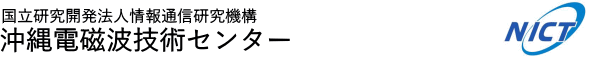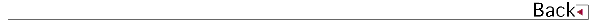# 2011\$BG/EY(B (2011.04\$B!A(B2012.03)

## \$B8&5fO@J8(B

• \$BBtED(B \$B2mMN(B,\$B4d:j(B \$B=S(B \$B0NL@(B,\$B;3:j(B \$B9d(B,\$B4d0f(B \$B9(FA(B,\$B@P0f(B \$B>;7{(B,\$B?eC+(B \$B9LJ?(B,\$BHDIt(B \$BIROB(B,\$B;3ED(B \$B@t(B,Transient Downslope Winds under the Influence of Stationary Lee Waves from the Zao Mountain Range,Journal of the Meteorological Society of Japan
• \$B4d0f(B \$B9(FA(B,\$BB<;3(B \$BBY7<(B,\$B@P0f(B \$B>;7{(B,\$B?eC+(B \$B9LJ?(B,\$BBgLn(B \$BM50l(B,\$B668}(B \$BB@0lO:(B,\$B%I%C%W%i!<%i%\$%@!<\$H%7!<%m%a!<%?\$K\$h\$j4QB,\$5\$l\$?3\$IwA0@~\$G\$N6/\$\$>e>:N.\$H\$=\$l\$K4XO"\$9\$kCOI=LL6aK5\$N9bG;EY%(%"%m%>%k\$N1tD>M"Aw(B,Strong Updraft at a Sea-Breeze Front and Associated Vertical Transport of Near-Surface Dense Aerosol Observed by Doppler Lidar and Ceilometer,Boundary-Layer Meteorology

## \$B3X=Q2r@b(B

• \$B@P0f(B \$B>;7{(B,\$B4d0f(B \$B9(FA(B,\$B?eC+(B \$B9LJ?(B,\$B%I%C%W%i!<%i%\$%@!<\$K\$h\$kIw4QB,\$H\$=\$N1~MQ(B,Doppler Lidar for Wind Measurement and Its Applications,\$B%l!<%6!<3X2q;o!V%l!<%6!<8&5f!W!!(B

## \$B<}O?O@J8(B

• \$B@P0f(B \$B>;7{(B,\$B?eC+(B \$B9LJ?(B,\$BJ!2,Bg3Y(B,\$B@P@nN4>M(B,Baron Philippe,\$BEDCfCR>O(B,\$B?9LnM&(B,\$BFbLn=\$(B,\$B4d0f(B \$B9(FA(B,\$B@DLZ(B \$BE/O:(B,\$BHDIt(B \$BIROB(B,\$B:4F#FF(B,Development of Coherent 2-\$B&L(Bm Differential Absorption and Wind Lidar with laser frequency offset locking technique and column-integrated CO2 measurement,16th Coherent Laser Radar Conference
• \$B?eC+(B \$B9LJ?(B,\$BHDIt(B \$BIROB(B,\$B@P0f(B \$B>;7{(B,\$B4d0f(B \$B9(FA(B,\$B@u0fOB90(B,\$B:4F#FF(B,\$BJ!2,Bg3Y(B,\$B@P@nN4>M(B,\$B2C@%DgFs(B,\$BDGL>E/CK(B,\$BIw\$HFs;@2=C:AG4QB,MQ\$N(B2\$B%_%/%m%s%l!<%6\$N3+H/(B,2-micron laser development for wind and CO2 observations,16\$B2s%3%R!<%l%s%H%l!<%6%l!<%@2q5D(B
• \$B>.ED(B \$BN=;R(B,\$B4d0f(B \$B9(FA(B,\$B0p3@(B \$B8|;j(B,\$B@P0f(B \$B>;7{(B,\$B:4F#(B \$B?82p(B,\$B4X_7(B \$B?.Li(B,\$B?eC+(B \$B9LJ?(B,\$BB<;3(B \$BBY7<(B,\$B%I%C%W%i!<%i%\$%@!<4QB,\$K4p\$E\$\$\$?ET;T0h\$NLk4V0BDj6-3&AXFbMpN.8=>](B,STRUCTURE OF STABLE NOCTURNAL BOUNDARY LAYER IN URBAN AREA DETECTED IN A DOPPLER LIDAR OBSERVATION,\$BBh(B56\$B2s?e9)3X9V1i2q(B
• \$B@nB<(B \$B@?<#(B,\$B2VEZ(B \$B90(B,\$B?yC+(B \$BLPIW(B,\$BCf@n(B \$B>!9-(B,\$B0f8}(B \$B=SIW(B,GPM/DPR ground validation super site in Okinawa,, Japan,The 28th International Symposium on Space Technology and Science
• \$BCf@n(B \$B>!9-(B,\$B2VEZ(B \$B90(B,\$B@nB<(B \$B@?<#(B,\$B?yC+(B \$BLPIW(B,\$B@6?e(B \$B<};J(B,\$BCfB<(B \$B7r<#(B,\$B@>@n(B \$B>-E5(B,\$BL1ED(B \$B@2Li(B,Multi-frequency rainfall observation for GPM/DPR algorithm development,The 28th International Symposium on Space Technology and Science

## \$B0lHL8}F,H/I=(B

• \$BBl@n!!E/B@O:(B,\$B?9K\!!>e=\\$KGk;T8+Eg<~JU3\$0h\$G4QB,\$5\$l\$?J?6QN.F09=B\$(B,\$BJ?@.(B23\$BG/EYL>8E20Bg3XCO5e?e=[4D8&5f%;%s%?!<8&5f=82q(B
• \$B?9K\>eN.0h\$N6&F18&5f(B,\$BJ?@.(B23\$BG/EYL>8E20Bg3XCO5e?e=[4D8&5f%;%s%?!<8&5f=82q(B
• \$B?yC+(B \$BLPIW(B,\$B>pJsDL?.8&5f5!9=(B \$B2-FlEE<'GH5;=Q%;%s%?!<\$N>R2p(B,Introduction of Okinawa Electromagnetic
• \$B?yC+(B \$BLPIW(B,\$B2VEZ(B \$B90(B,\$B@nB<(B \$B@?<#(B,\$B2-FlK\Eg@>4_0h\$K\$*\$1\$kig5\$O0O"B34QB,(B,\$BF|K\5\$>]3X2q!!=U5(Bg2q(B
• \$B?yC+(B \$BLPIW(B,\$B@nB<(B \$B@?<#(B,\$B2VEZ(B \$B90(B,\$BJ,;67?3\$MN%l!<%@5;=Q3+H/0F(B,\$BF|K\CO5eOG@12J3XO"9g(B2011\$BG/Bg2q(B
• \$B?yC+(B \$BLPIW(B,\$B2VEZ(B \$B90(B,\$B@nB<(B \$B@?<#(B,\$BCf@n(B \$B>!9-(B,GPM\$B!&(BDPR\$B50F;>e9;@5MQ%l!<%@9;@54o\$N3+H/(B,Development of Radar Calibrators for the Dual-frequency Precipitation Radar(DPR) installed on the Global Precipitation Measurement(GPM) primary satellite,International Symposium on Space Technology and Science (ISTS)\$B!!(B
• \$B?yC+(B \$BLPIW(B,\$B4d0f(B \$B9(FA(B,\$B2VEZ(B \$B90(B,\$B%5%s%4>L!&1h4_3\$0h4QB,MQ%7!<%m%a!<%?2sE>Bf\$N@=:n(B,Hy-ARC\$B!>(BNICT\$B8&5f=82q(B
• \$B@nB<(B \$B@?<#(B,\$B?yC+(B \$BLPIW(B,\$B2VEZ(B \$B90(B,\$BCf@n(B \$B>!9-(B,DEVELOPMENT OF A NEW BISTATIC DOPPLER MEASUREMENT SYSTEM AND ITS FIELD TEST IN OKINAWA,2011 IEEE International Geoscience and Remote Sensing Symposium
• \$B@nB<(B \$B@?<#(B,\$B?yC+(B \$BLPIW(B,\$B2VEZ(B \$B90(B,\$BCf@n(B \$B>!9-(B,\$B2-FlJPGH9_1+%l!<%@(BCOBRA\$B\$K\$h\$k2~NI7?%P%\$%9%?%F%#%C%/;n834QB,(B,\$BF|K\CO5eOG@12J3XO"9g(B2011\$BG/Bg2q(B
• \$B@nB<(B \$B@?<#(B,\$B?yC+(B \$BLPIW(B,\$B2VEZ(B \$B90(B,\$BCf@n(B \$B>!9-(B,\$B2-FlJPGH9_1+%l!<%@(BCOBRA \$B\$rMQ\$\$\$?2~NI7?%P%\$%9%?%F%#%C%/4QB,%7%9%F%`\$NZ]3X2q!!=U5(Bg2q(B
• \$B:4F#(B \$B?82p(B,\$B2VEZ(B \$B90(B,\$B@nB<(B \$B@?<#(B,\$B?yC+(B \$BLPIW(B,\$BCf@n(B \$B>!9-(B,\$BB<;3(B \$BBY7<(B,\$B8EK\(B \$B=_0l(B,\$BDEED(B \$BIRN4(B,400MHz\$BBS(BWPR/RASS\$B\$K\$h\$k2-Fl0!G.BS0h\$NIwB.\$*\$h\$S5\$29%W%m%U%!%\$%k\$NDj>o4QB,\$N8=>u\$H2]Bj(B,\$BF|K\5\$>]3X2q(B2011\$BG/EY=U5(Bg2q(B
• \$BCf@n(B \$B>!9-(B,\$B2VEZ(B \$B90(B,\$B@nB<(B \$B@?<#(B,\$B?yC+(B \$BLPIW(B,\$B@6?e(B \$B<};J(B,\$BCfB<(B \$B7r<#(B,\$B@>@n(B \$B>-E5(B,\$BL1ED(B \$B@2Li(B,Multi-frequency rainfall measurements for GPM/DPR algorithm development,AOGS2011
• \$BCf@n(B \$B>!9-(B,Masanori Nishikawa,Kenji Nakamura,Mariko Oue,\$B2VEZ(B \$B90(B,Shuji Shimizu,Haruya Minda,Field Campaign for GPM/DPR Ground Validation using the Dual Ka-band Radar system,PMM 2011
• \$BCf@n(B \$B>!9-(B,\$B@>@n(B \$B>-E5(B,\$BCfB<(B \$B7r<#(B,\$B2VEZ(B \$B90(B,\$BL1ED(B \$B@2Li(B,\$B>.D.(B \$B7r0l(B,\$B@6?e(B \$B<};J(B,\$B2-(B \$BM};R(B,GPM GV Activity in Japan -Dual Ka-radar campaign observation- ,The 3rd GPM Asia Workshop on Precipitation Date Application Technique
• \$B@P0f(B \$B>;7{(B,\$B?eC+(B \$B9LJ?(B,Baron Philippe,\$B4d0f(B \$B9(FA(B,\$B>.ED(B \$BN=;R(B,\$BHDIt(B \$BIROB(B,\$B>.;3!!?p]3X2q(B
• \$B@P0f(B \$B>;7{(B,\$B>.;3?p.EDN=;R(B,\$BJ!2,Bg3Y(B,\$B@P@nN4>M(B,\$BHDIt(B \$BIROB(B,\$B:4F#(B \$BFF(B,Recent results of ground-based CO2 experiment at NICT: atmospheric and hard target returns,5th International Workshop on CO2/CH4 DIAL remote sensing
• \$B@P0f(B \$B>;7{(B,Baron Philippe,\$BHDIt(B \$BIROB(B,\$B?eC+(B \$B9LJ?(B,\$B4d0f(B \$B9(FA(B,\$B>.ED(B \$BN=;R(B,\$B>.;3(B \$B?pZ
• \$B?yC+(B \$BLPIW(B,\$B4d0f(B \$B9(FA(B,\$B2VEZ(B \$B90(B,\$B@nB<(B \$B@?<#(B,\$BJ,;67?3\$MN%l!<%@
• \$B@nH*(B \$BBsLp(B,\$B4d0f(B \$B9(FA(B,\$B@%8E(B \$B90(B,\$B@FF#(B \$BOBM:(B,\$B@P0f(B \$B>;7{(B,\$B?eC+(B \$B9LJ?(B,\$B%I%C%W%i!<%i%\$%@!<4QB,%G!<%?F12=]3X2q(B2011\$BG/EY=)5(Bg2q(B
• \$B@nB<(B \$B@?<#(B,\$B?yC+(B \$BLPIW(B,\$B4d0f(B \$B9(FA(B,\$B2VEZ(B \$B90(B,\$BM?Fa9q\$K\$*\$1\$k3\$MN%l!<%@\$N%P%\$%9%?%F%#%C%/
• \$B@nB<(B \$B@?<#(B,\$B?yC+(B \$BLPIW(B,\$B4d0f(B \$B9(FA(B,\$B2VEZ(B \$B90(B,\$BM?Fa9q\$K\$*\$1\$k3\$MN%l!<%@!&%P%\$%9%?%F%#%C%/]!&5\$8u!&3\$>]\$N4QB,E*8&5f\$K4X\$9\$k6&F18&5f=82q(B
• \$B@nB<(B \$B@?<#(B,\$B?yC+(B \$BLPIW(B,\$B4d0f(B \$B9(FA(B,\$BCf@n(B \$B>!9-(B,\$B2VEZ(B \$B90(B,AN IMPROVED BISTATIC DOPPLER MEASUREMENT SYSTEM WITH REDUCED CONTAMINATION BY SIDELOBE ECHOES,35th Conference on Radar Meteorology
• \$B4d0f(B \$B9(FA(B,\$BB<;3(B \$BBY7<(B,\$B4X_7(B \$B?.Li(B,\$BCf@n(B \$B>!9-(B,\$B>.ED(B \$BN=;R(B,\$B@P0f(B \$B>;7{(B,\$B?eC+(B \$B9LJ?(B,\$B%I%C%W%i!<%i%\$%@!<\$H%&%#%s%I%W%m%U%!%\$%i\$K\$h\$j4QB,\$5\$l\$?(B2010\$BG/(B7\$B7n(B5\$BF|\$N6ICOE*Bg1+(B,\$BF|K\5\$>]3X2q(B2011\$BG/EY=U5(Bg2q(B
• \$B4d0f(B \$B9(FA(B,\$B?yC+(B \$BLPIW(B,\$B2VEZ(B \$B90(B,\$B%l!<%6%j%b!<%H%;%s%7%s%0\$K\$h\$k%5%s%4>L!&1h4_0h\$NBg5\$JQF0\$N4QB,(B,\$B2-Fl\$N5\$>]!&5\$8u!&3\$>]\$N4QB,E*8&5f\$K4X\$9\$k6&F18&5f=82q(B
• \$B4d0f(B \$B9(FA(B,\$BB<;3(B \$BBY7<(B,\$B4X_7(B \$B?.Li(B,\$BCf@n(B \$B>!9-(B,\$B>.ED(B \$BN=;R(B,\$B@P0f(B \$B>;7{(B,\$B?eC+(B \$B9LJ?(B,2010\$BG/(B7\$B7n(B5\$BF|\$N6ICOE*Bg1+;~\$N9_?e0hFb30\$NIwB.>l(B,Doppler lidar and wind profiler observation of a localized heavy rainfall event on 5 July 2010,\$BF|K\CO5eOG@12J3XO"9g(B2011\$BG/Bg2q(B
• \$B>.ED(B \$BN=;R(B,\$B4d0f(B \$B9(FA(B,\$B:4F#(B \$B?82p(B,\$B4X_7(B \$B?.Li(B,\$B@P0f(B \$B>;7{(B,\$B?eC+(B \$B9LJ?(B,\$BB<;3(B \$BBY7<(B,\$BEl5~ET;T0h\$K\$*\$1\$kLk4V6-3&AXFbMpN.8=>](B,Structure of nocturnal turbulent mixing in Tokyo,\$BF|K\CO5eOG@12J3XO"9g(B2011\$BG/Bg2q(B
• \$B>.ED(B \$BN=;R(B,\$B4d0f(B \$B9(FA(B,\$B:4F#(B \$B?82p(B,\$B4X_7(B \$B?.Li(B,\$B@P0f(B \$B>;7{(B,\$B?eC+(B \$B9LJ?(B,\$BB<;3(B \$BBY7<(B,\$BEl5~ET;T0h\$K\$*\$1\$kLk4V\$N1tD>N.>qMp(B,\$BF|K\5\$>]3X2q!!=U5(Bg2q(B
• \$B0B0f(B \$B85><(B,\$B@P0f(B \$B>;7{(B,\$B4d0f(B \$B9(FA(B,\$BB<;3(B \$BBY7<(B,\$B?eC+(B \$B9LJ?(B,\$B>.EDN=;R(B,NICT\$B\$K\$*\$1\$k%I%C%W%i!<%i%\$%@!<3+H/\$H:#8e\$N7W2h(B,\$BBh#12s6KC<5\$>]\$K4X\$9\$k%o!<%/%7%g%C%W(B
• \$B:4F#(B \$B?82p(B,\$B2VEZ(B \$B90(B,\$B@nB<(B \$B@?<#(B,\$B4d0f(B \$B9(FA(B,\$BB<(B,\$B1:DM(B \$B@6Jv(B,COBRA\$B%G!<%?\$N>pJs2=\$H#3
• \$BCf@n(B \$B>!9-(B,\$B2VEZ(B \$B90(B,\$B@nB<(B \$B@?<#(B,\$B4d0f(B \$B9(FA(B,\$B?yC+(B \$BLPIW(B,COBRA\$B\$rMQ\$\$\$?(BTRMM/PR V7\$B\$NI>2A(B,\$BJ?@.(B23\$BG/EYL>8E20Bg3XCO5e?e=[4D8&5f%;%s%?!<8&5f=82q!VBg5\$3\$MN8=>]\$N%j%b!<%H%;%s%7%s%0%7%9%F%`5;=Q\$N3+H/\$K4X\$9\$k6&F18&5f=82q!W(B

## 1990\$B!A(B1991\$B!d(B1992\$B!d(B1993\$B!d(B1994\$B!d(B1995\$B!d(B1996\$B!d(B1997\$B!d(B1998\$B!d(B 1999\$B!d(B2000\$B!d(B2001\$B!d(B2002\$B!d(B2003\$B!d(B2004\$B!d(B2005\$B!d(B2006\$B!d(B2007\$B!d(B 2008\$B!d(B2009\$B!d(B2010\$B!d(B2011\$B!d(B2012\$B!d(B2013\$B!d(B2014\$B!d(B2015\$B!d(B2016\$B!d(B2017